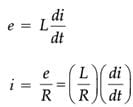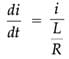# Assertion & Reason Test: Alternating Current - 1

## 15 Questions MCQ Test Physics Class 12 | Assertion & Reason Test: Alternating Current - 1

Description
Attempt Assertion & Reason Test: Alternating Current - 1 | 15 questions in 30 minutes | Mock test for NEET preparation | Free important questions MCQ to study Physics Class 12 for NEET Exam | Download free PDF with solutions
QUESTION: 1

### Directions : In the following questions, A statement of Assertion (A) is followed by a statement of Reason (R). Mark the correct choice as.Assertion (A): An alternating current does not show any magnetic effect.Reason (R): Alternating current changes direction with time.

Solution: Current or moving charged particle creates magnetic field irrespective of direct current or alternating current. So assertion is false. Alternating current changes direction with time. So, the reason is true, but cannot explain the assertion.
QUESTION: 2

### Directions : In the following questions, A statement of Assertion (A) is followed by a statement of Reason (R). Mark the correct choice as.Assertion (A): VRMS value of an alternating voltage V = 4√2 sin 314t is 4 volt.Reason (R): Peak value of the alternating voltage is 4√2 volt.

Solution: Given alternating voltage V = 4√2 sin 314t. Where peak value = V0 = 4√2 volt. VRMS = V0/√2 = 4 volt. Hence both assertion and reason both are true. But the reason does not properly explain the assertion.
QUESTION: 3

### Directions : In the following questions, A statement of Assertion (A) is followed by a statement of Reason (R). Mark the correct choice as.Assertion (A): The dimension of L/R is time.Reason (R): Time constant (L/R) should be increased to reduce the rate of increase of current through a solenoid.

Solution: For a solenoid, the magnitude of induced emfIn left hand side of the above equation, denominator is time. So, in right hand side, the denominator should be time. So, dimension of L/R is time. So, the assertion is true. If L/R increases, di/dt decreases. So, reason is also true. But reason cannot properly explain the assertion.

QUESTION: 4

Directions : In the following questions, A statement of Assertion (A) is followed by a statement of Reason (R). Mark the correct choice as.

Assertion (A): When capacitive reactance is less than the inductive reactance in a series LCR circuit, e.m.f. leads the current.

Reason (R): The angle by which alternating voltage leads the alternating current in series RLC circuit is given by tan φ = XL - XC /R.

Solution: The angle by which alternating voltage leads the alternating current in series RLC circuit is given by tan φ = XL - XC /R. If XC < XL, then tan j is positive. φ is also positive. So, e.m.f. leads the current. Assertion and reason both are true. Reason properly explains the assertion.
QUESTION: 5

Directions : In the following questions, A statement of Assertion (A) is followed by a statement of Reason (R). Mark the correct choice as.

Assertion (A): Principle of operation of AC generator is electromagnetic induction.

Reason (R): Resistance offered by inductor for AC is zero.

Solution: Principle of operation of AC generator is electromagnetic induction. The assertion is true. Resistance offered by inductor = 2πfL. For AC, f ≠ 0. So, 2πfL ≠ 0. So, the reason is false.
QUESTION: 6

Directions : In the following questions, A statement of Assertion (A) is followed by a statement of Reason (R). Mark the correct choice as.

Assertion (A): A transformer does not work on DC.

Reason (R): DC neither change direction nor magnitude.

Solution: Transformer has two coils. If current fluctuates in one coil, e.m.f. is induced in the other coil. For DC supply current does not change, so there is no induced e.m.f. Hence both assertion and reason are true and reason explains the assertion.
QUESTION: 7

Directions : In the following questions, A statement of Assertion (A) is followed by a statement of Reason (R). Mark the correct choice as.

Assertion: In series LCR circuit resonance can take place.

Reason: Resonance takes place if inductance and capacitive reactances are equal and opposite.

Solution: At resonant frequency, XL=XC \ Z = R (minimum) there for current in the circuit is maximum.
QUESTION: 8

Directions : In the following questions, A statement of Assertion (A) is followed by a statement of Reason (R). Mark the correct choice as.

Assertion: Capacitor serves as a block for dc and offers an easy path to ac.

Reason: Capacitive reactance is inversely proportional to frequency.

Solution: The capacitive reactance of capacitor is given by XC = 1/ωC = 1/2πfC| So this is infinite for dc (f = 0) and has a very small value for ac. Therefore a capacitor blocks dc.
QUESTION: 9

Directions : In the following questions, A statement of Assertion (A) is followed by a statement of Reason (R). Mark the correct choice as.

Assertion: Chock coil is preferred over a resistor to adjust current in an ac circuit.

Reason: Power factor for inductance is zero.

Solution: If resistor is used in controlling ac supply, electrical energy will be wasted in the form of heat energy across the resistance wire. However, ac supply can be controlled with choke without any wastage of energy. This is because, power factor (cosf) for resistance is unity and is zero for an inductance. [P = EI cosf].
QUESTION: 10

Directions : In the following questions, A statement of Assertion (A) is followed by a statement of Reason (R). Mark the correct choice as.

Assertion: A bulb connected in series with a solenoid is connected to ac source. If a soft iron core is introduced in the solenoid, the bulb will glow brighter.

Reason: On introducing soft iron core in the solenoid, the inductance increases.

Solution: On introducing soft iron core, the bulb will glow dimmer. This is because on introducing soft iron core in the solenoid, its inductance L increases, the inductive reactance, XLL increases and hence the current through the bulb decreases.
QUESTION: 11

Directions : In the following questions, A statement of Assertion (A) is followed by a statement of Reason (R). Mark the correct choice as.

Assertion: The dc and ac both can be measured by a hot wire instrument.

Reason: The hot wire instrument is based on the principle of magnetic effect of current.

Solution: Both ac and dc produce heat, which is proportional to square of the current. The reversal of direction of current in ac is immaterial so far as production of heat is concerned.
QUESTION: 12

Directions : In the following questions, A statement of Assertion (A) is followed by a statement of Reason (R). Mark the correct choice as.

Assertion: Average value of ac over a complete cycle is always zero.

Reason: Average value of ac is always defined over half cycle.

Solution: The mean average value of alternating current (or emf) during a half, cycle is given by Im = 0.636 I0 (or Em = 0.636 E0) During the next half cycle, the mean value of ac will be equal in magnitude but opposite in direction. For this reason the average value of ac over a complete cycle is always zero. So the average value is always defined over a half cycle of ac.
QUESTION: 13

Directions : In the following questions, A statement of Assertion (A) is followed by a statement of Reason (R). Mark the correct choice as.

Assertion: When ac circuit contain resistor only, its power is minimum.

Reason: Power of a circuit is independent of phase angle.

Solution: The power of a ac circuit is given by P=EI cosφ where cosf is power factor and f is phase angle. In case of circuit containing resistance only, phase angle is zero and power factor is equal to one. Therefore power is maximum in case of circuit containing resistor only.
QUESTION: 14

Directions : In the following questions, A statement of Assertion (A) is followed by a statement of Reason (R). Mark the correct choice as.

Assertion: A capacitor of suitable capacitance can be used in an ac circuit in place of the choke coil.

Reason: A capacitor blocks dc and allows ac only.

Solution: We can use a capacitor of suitable capacitance as a chock coil, because average power consumed per cycle in an ideal capacitor is zero. Therefore, like a choke coil, a condenser can reduce ac without power dissipation.
QUESTION: 15

Directions : In the following questions, A statement of Assertion (A) is followed by a statement of Reason (R). Mark the correct choice as.

Assertion: Average power in series LCR ac circuit is maximum at resonance.

Reason: At the resonance circuit is purely resistive.

Solution: At resonance, the impedance of the circuit is reduced to the minimum of Z=RΩ, by definition, as reactance of inductor and capacitor are equal in magnitude. Hence the current is the circuit is maximumUse Code STAYHOME200 and get INR 200 additional OFF Use Coupon Code